1. Determine the nature of roots of each of the following equations
1.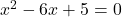2.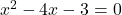3.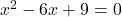4.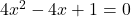5.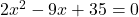6.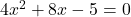2. For what values of p will the equation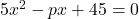?

3. if the equation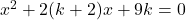has equal roots, find k.

4. For what value of a will the equation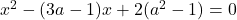have equal roots?

5. If the roots of the equation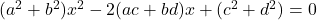are equal, then show that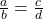6. Show that the roots of the equation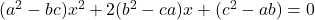will be equal, if either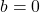or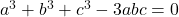7. If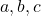are rational and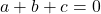, show that the roots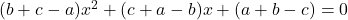are rational.

8. Prove that the roots of the equation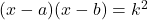are real for all values of k.
9. Show that the roots of the equation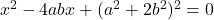are imaginary.
10. If the roots of the quadratic equation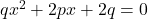are real and unequal, prove that the roots of the equation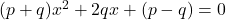are imaginary